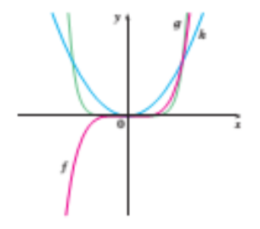1
83
views
3c
Problem

Problem 3c

Chapter 1: Functions and ModelsTextbook ExpertVerified Tutor
13 Oct 2021

Given information

The given function is .Step-by-step explanation

Step 1.

The function matches the f plot in the graph.

It is a cubic function.  The cube of a negative number is negative.

Hence, a cubic function plot comes in the third and 1st quadrant.